# Go Forth and Generalise

##### Age 11 to 14

Published 2004 Revised 2012

We are often presented with mathematical problems where we are asked to identify a pattern that is generated by a situation and then give a general rule for what we see.

This article is about the step that we often miss in this process: explaining why the pattern works and why the general rule can be applied to the original situation. That is, trying to explain how the mathematics represents the picture.

There are many problems on the NRICH site that ask students to find a general rule, and it is often the case that the solutions we receive finish with a mathematical formula that has come from spotting a pattern...

For example, in the problem 1 Step 2 Step:

Liam's house has a staircase with 12 steps. He can go down the steps one at a time or two at time.

For example: He could go down 1 step, then 1 step, then 2 steps, then 2, 2, 1, 1, 1, 1.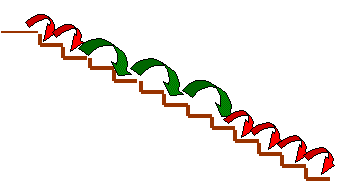In how many different ways can Liam go down the 12 steps, taking one or two steps at a time?

The solution shows that the number of ways you can move down flights of stairs of differing lengths forms a sequence:

1, 2, 3, 5, 8, 13 ... and the solvers identify this as the beginning of the Fibonacci sequence:

1 + 2 = 3

2 + 3 = 5

3 + 5 = 8

5 + 8 = 13

and so were able to predict the 12th number in the sequence.

But how do we know that the situation introduced in the problem will continue to obey this rule and that it is reasonable to just extend the pattern to the 12th number? It might just break down.

The only way to be sure is to explain the mathematics, in this case the fact that you add the last two numbers in the sequence to get the next number. How does/can this reflect what is happening? If you cannot make the connection you cannot be sure that the general rule always works.

Let's look at another example:

This pattern of six squares is made from 19 matches. How many matches would you need to make a pattern 20, 100 or N squares long?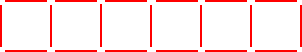You might start to answer this question by drawing the above pattern like this: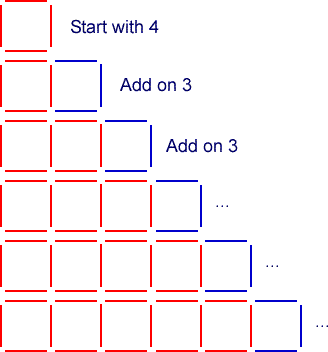In this case you might come to a total of 19 matches by using a calculation that reflects your construction, that is 4 + 5 x 3 = 19

In which case you would have noticed that you start with a square then add three matches at a time and because you need a total of 6 squares you need 5 (one less than 6) lots of three.

This might lead you to say that for a pattern 20 squares long you would need

4 + 19 x 3 = 61 matches

So for a pattern 100 long it would be: 4 + 99 x 3 = 301

And for one that is N long it would be: 4 + 3(N-1), which can be simplified to 4 + 3N - 3 = 3N + 1

But of course you might have drawn it this way: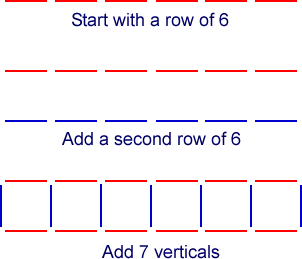In which case your calculations might have gone as follows:

Starting with a row of 6, then a second row of 6 and then 7 (one more than the number of squares) = 2 x 6 +7 = 19

This might lead you to say that for a pattern 20 squares long you would need 2 x 20 + 21= 61 matches

So for a pattern 100 long it would be: 2 x 100 + 101 = 301

And for one that is N long it would be 2N + N + 1, which can be simplified to 3N + 1.

Although you approached creating the pattern in different ways and the basis of your generalisation was different in both cases you have ended up with the same general rule: 3N + 1.

There is of course the added value of being able to use the notion of simplification of terms to support the ability to show the equivalence of the two methods. A very useful opportunity to include an aspect of mathematics we often learn as a skill with little apparent value.

But, of course we now have a general rule which has come out of the structure of the problem, which is 3N + 1.

Reflecting back can we create a picture of how to construct the original six squares using this generalisation? i.e. 3 x 6 + 1. Well it might look like this: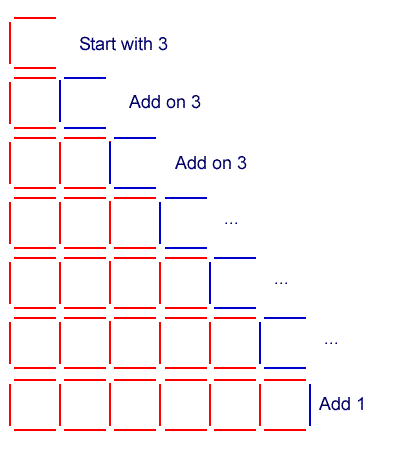Now that feels convincing and I feel confident that I was justified in generalising.

So are we justified in suggesting that the 1 step 2 step problem will always generate Fibonacci numbers?

If I need to go down 5 steps I can either go down the first 3 steps (in 3 ways) and finish with a double step, or go down the first 4 steps (in 5 ways) and finish with a single step. So the number of ways of going down 5 steps will be the sum of the number of ways of going down 3 steps plus the number of ways of going down 4 steps.

Likewise, if I need to go down 20 steps I can either go down the first 18 steps and finish with a double step, or go down the first 19 steps and finish with a single step. So the number of ways of going down 20 steps will be the sum of the number of ways of going down 18 steps plus the number of ways of going down 19 steps.

It looks like we are justified in using Fibonacci numbers to predict the total number of ways of going down the 12 steps.

Spotting patterns can be an important first step - understanding why the pattern works and explaining why it is appropriate to generalise is the next step, and often the most interesting and important.